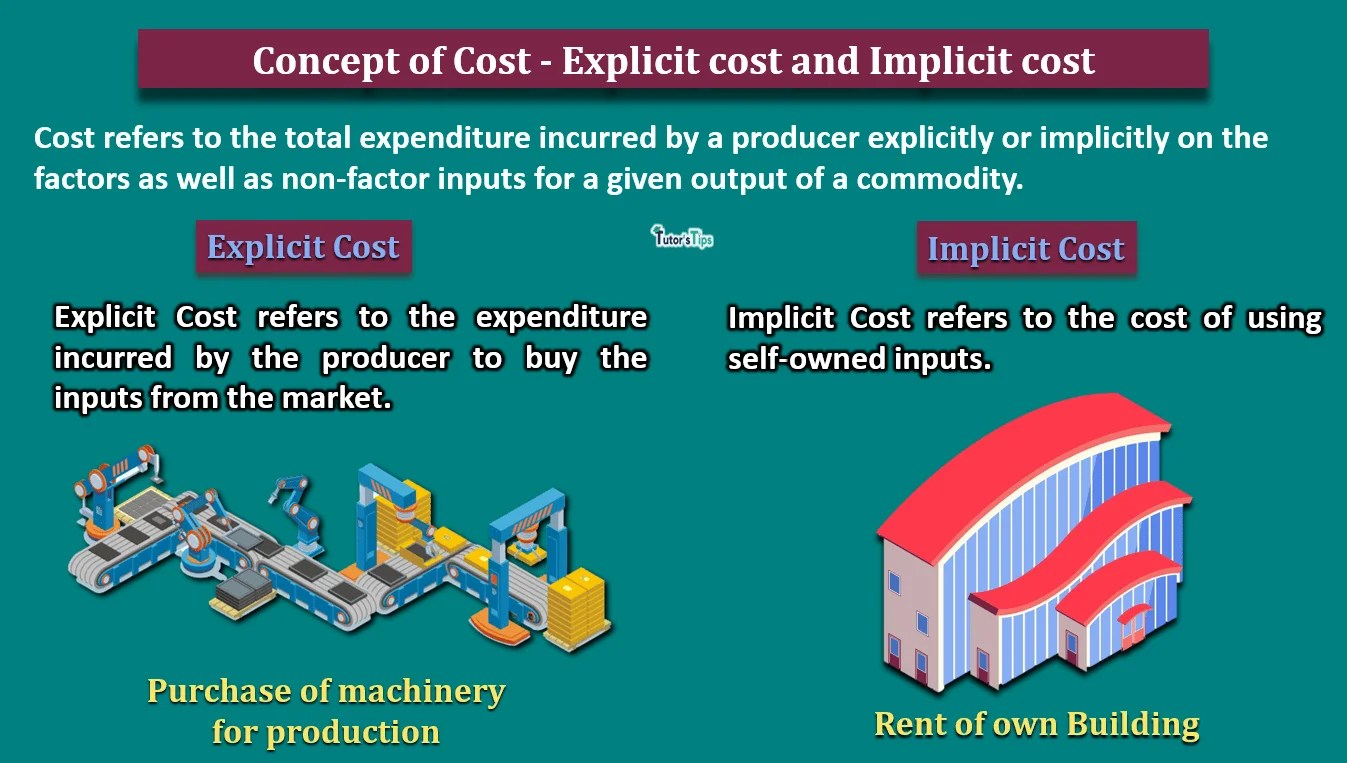# Concept of Cost – Implicit and Explicit CostThe concept of cost defines the total expenditure incurred by the producer while producing an output.

## Concept of Cost:

The concept of cost refers to the total expenditure incurred by a producer explicitly or implicitly on the factors as well as non-factor inputs for a given output of a commodity.

It is the measurement of the number of resources used in the production of goods and rendering of services. When the goods are produced or the services are rendered, various expenses have to be incurred including wages to labour, rent to the landlord,  purchase of raw material and other expenses. Thus, the sum total of these expenses plus a normal profit margin expected by the producer is regarded as the total cost of production.

According to the Institute of Cost and Work Accountants,

“Cost implies measurement in monetary terms of the number of resources used for the purpose of production of goods and rendering of services.”

In other words, the cost can be defined as the amount of money for which any commodity or service is generated.

## Explicit Cost :

It refers to the expenditure incurred by the producer to buy the inputs from the market. For example, the purchase of raw material, labour from the market etc.

It can be measured as the cash payments that a firm makes to others for the purchase of inputs in the market. These are also known as Out of Pocket costs. The explicit costs can be determined easily in the market and require cash physical outflow of cash. Therefore, these are the costs which are considered while calculating accounting profit as well as economic profit.

## Implicit Cost :

It refers to the cost of using self-owned inputs. Therefore, the implicit cost refers to the estimated expenditure on the use of self-owned inputs. For example, estimated rent on owner’s building and estimated wages to family labour etc.

It is measured as imputed or estimated costs of self-owned and self-employed resources. Hence, implicit costs can be referred to as opportunity costs or imputed costs. The measurement or calculation of implicit costs is difficult as the producer doesn’t have to pay it in reality. Thus, these costs are considered only while calculating economic profit.

In economics, the total cost is estimated as the sum total of these both elements which are implicit cost and explicit cost.

Total Cost = Explicit Cost + Implicit Cost

## Illustration :

Suppose, a person left a job where he gets Rs.40000 per month and started undertaking the production of garments at his own premises where he can get Rs.60000 as rent. He pays Rs. 100000 for the purchase of inputs. Thus, the total cost would be:

Total Cost = Explicit Cost + Implicit Cost

Explicit cost = Payment to others for purchase of inputs = Rs.100000

Implicit Cost = Value of self-owned inputs = His own services + Imputed Rent = Rs.(40000+60000) = Rs.100000

Therefore,

Total Cost = 100000+100000

= Rs 200000